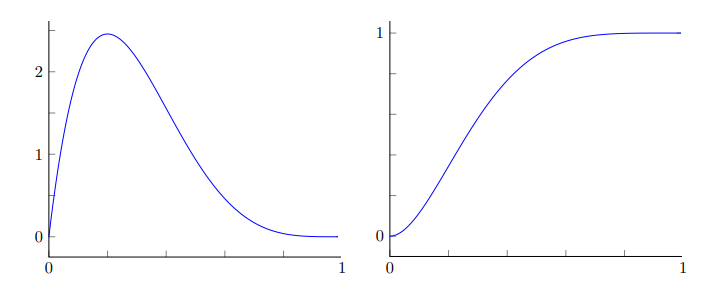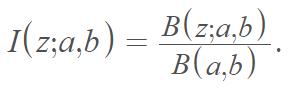# Regularized Incomplete Beta Function

The regularized incomplete beta function (also called the regularized beta function) is widely used in statistics and some areas of physics as the cumulative distribution function of the beta distribution . It represent the CDF for a random number Y that obeys the beta distribution .Left: PDF of the beta distribution (a = 2, b = 5) Right: CDF (regularized incomplete beta function) of the same distribution.

The regularized incomplete beta function is used when it’s inconvenient to calculate the incomplete beta function on its own. The “regularized” version divides this function by the complete beta function , hence another alternate name — the incomplete beta function ratio .

## Formula for the Regularized Incomplete Beta Function

The regularized incomplete beta function is defined in terms of the incomplete beta function B(z; a, b) — also called the Euler integral — and the complete beta function b (a. b) :The basic definition shown above cannot be used for nonpositive integers a or b, as this will lead to an indeterminate expression. For nonpositive integers, you may need to use a more complete definition such as :## References

 Conte, E. On a Simple Derivation of the Effect of Repeated Measurements on Quantum Unstable Systems by Using the Regularized Incomplete Beta Function. Adv. Studies Theor. Phys., Vol. 6, 2012, no. 25, 1207 – 1213.